Next: The Sun Up: Geometric Planetary Orbit Models Previous: Model of Ptolemy

# Model of Copernicus

Copernicus' geometric model of a heliocentric planetary orbit is illustrated in Fig. 20. The planetrotates on a circular epicycle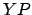whose centermoves around the sun on the eccentric circle(only half of which is shown). The diameter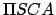is the effective major axis of the orbit, where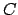is the geometric center of circle, and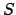the fixed position of the sun. Whenis atorthe planet is at its perihelion or aphelion points, respectively. The radiusof circleis the effective major radius,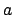, of the orbit. The distance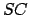is equal to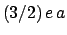, where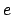is the orbit's effective eccentricity. Moreover, the radius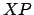of the epicycle is equal to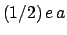. The angle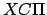is identified with the mean anomaly,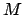, and increases linearly in time. In other words, as seen from, the center of the epicyclemoves uniformly around circlein a counterclockwise direction. The angle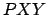, where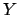is point at whichproduced meets the epicycle, is equal to the mean anomaly. In other words, the planetmoves uniformly around the epicycle, in an counterclockwise direction, at twice the speed that pointmoves around circle. Finally,is the radial distance,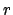, of the planet from the sun, and angleis the planet's true anomaly,.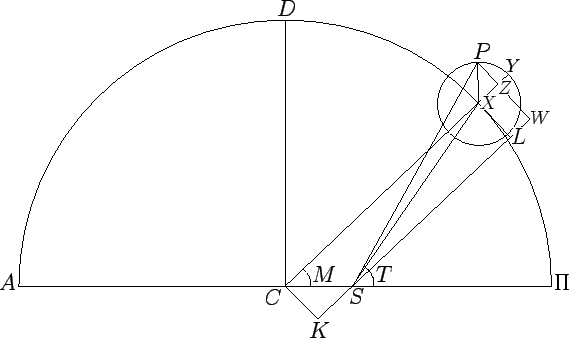Let us draw the straight-line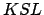parallel to, and passing through point, and then complete the rectangle. Simple geometry reveals that,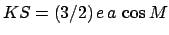, and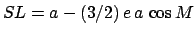. Letbe drawn normal to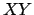, and let it meetproduced at point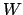. Simple geometry reveals that,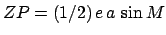, and. It follows that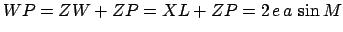, and. Moreover,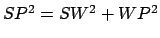, which implies that(91)

Now,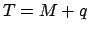, where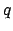is angle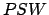. However,(92)

Finally, expanding the previous two equations to second-order in the small parameter, we obtain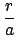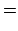(93)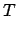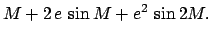(94)

It can be seen, by comparison with Eqs. (81)-(82) and (89)-(90), that, as is the case for Ptolemy's model, both the relative radial distance,, and the true anomaly,, in Copernicus' geometric model of a heliocentric planetary orbit only deviate from those in the (correct) Keplerian model to second-order in. However, the deviation in the Ptolemaic model is slightly smaller than that in the Copernican model. To be more exact, the maximum deviation inis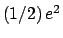in the former model, andin the latter. On the other hand, the maximum deviation inis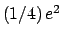in both models.Next: The Sun Up: Geometric Planetary Orbit Models Previous: Model of Ptolemy
Richard Fitzpatrick 2010-07-21